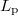﻿

### On simultaneous approximation

#### Abstract

In this paper first we give two different definitions for best simultaneous$%L_{\text{p }}$ approximation to$n$ functions and study the relation betweenbest simultaneous approximation and best$L_{\text{p }}$approximations tothe arithmetic mean of$n$ functions. In addition we consider the definitionand the theorem about the simultaneous approximation to$n$ ($n$ odd)functions in the ''sum'' norm .

DOI Code: 10.1285/i15900932v21n1p71

Keywords: Approximation; Simultaneous; Banach space

Classification: 41A28

Full Text: PDFThis work is licensed under a Creative Commons Attribuzione - Non commerciale - Non opere derivate 3.0 Italia License.